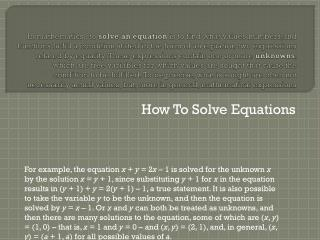DownloadDownload PresentationHow To Solve Equations

# How To Solve Equations

Download Presentation## How To Solve Equations

- - - - - - - - - - - - - - - - - - - - - - - - - - - E N D - - - - - - - - - - - - - - - - - - - - - - - - - - -
##### Presentation Transcript

1. In mathematics , to solve an equation is to find what values numbers,and functions fulfill a condition stated in the form of an equation two expressions related by equality. These expressions contain one or more unknowns, which are free variables for which values are sought that cause the condition to be fulfilled. To be precise, what is sought are often not necessarily actual values, but, more in general, mathematical expressions How To Solve Equations For example, the equation x + y = 2x – 1 is solved for the unknown x by the solution x = y + 1, since substituting y + 1 for x in the equation results in (y + 1) + y = 2(y + 1) – 1, a true statement. It is also possible to take the variable y to be the unknown, and then the equation is solved by y = x – 1. Or x and y can both be treated as unknowns, and then there are many solutions to the equation, some of which are (x, y) = (1, 0) – that is, x = 1 and y = 0 – and (x, y) = (2, 1), and, in general, (x, y) = (a + 1, a) for all possible values of a.